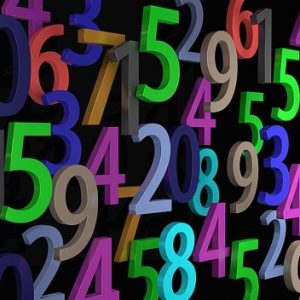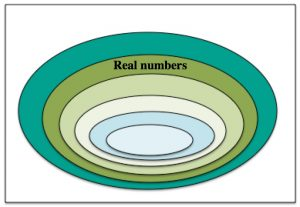How quickly can you find me?

I am a wholenumber less than 1000.

The product of my digits is 225.

I am not a multiple of 5.

Am I a multiple of 13?

Am I real? Am I rational? Am I irrational?

Now divide me by 11. Is the answer real? Is it rational?

Now multiply me by pi. Is the answer real? Is it rational?Copy this Venn diagram and label the following sets of numbers:

Integers, Natural numbers, Rational numbers, Whole numbers, Complex numbers, All numbers.

Click here for the Notes for Teachers.

Tagged with: